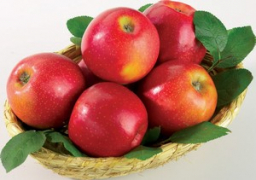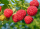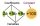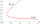Sadie picked up fallen apples from an apple tree in her yard. She picked up 14 green ones, 16 that were changing from green to red, and 18 that were red. What fraction in simplest form names the green apples?

x =  0.625

### Step-by-step explanation:

$x=\frac{14+16}{14+16+18}=\frac{5}{8}=0.625$Did you find an error or inaccuracy? Feel free to write us. Thank you!Tips to related online calculators
Need help to calculate sum, simplify or multiply fractions? Try our fraction calculator.

## Related math problems and questions:

• Simplest form of a fractionWhich one of the following fraction after reducing in simplest form is not equal to 3/2? a) 15/20 b) 12/8 c) 27/18 d) 6/4Sadie practiced her spelling words for 3/4 of an hour, and Max practiced his spelling words for 5/12 of an hour. In the simplest form, how much longer did Sadie practice than Max?
• Simplest form 3What fraction is 15 of 35 in simplest form?
• Apples 14Maya bought 18 apples. She used 50% of the apples to make apple pies, and 1/3 of the apples were mixed with other fruits to make the salad. How many apples were left?
• Guess a fractionTom was asked to guess a fraction. The sum of 1/2 the numerator and 1/3 of its denominator is 30. If Tom subtracts 36 from its denominator, the fraction becomes 1/3. What is the fraction that Tom was asked to guess? (Leave your answer in simplest form)
• How many 15How many six-eights are there in 18? Which of the following would you choose to solve this question? 18 x 6/8 or 18 : 6/8
• CarrieCarrie picked 2/5 of the raspberries from the garden, and Robin picked some too.  When they were finished, 1/3 of the raspberries still needed to be picked.  What fraction of the raspberries did Robin pick? Use pictures, numbers or words and write your fi
• In the cafeteriaThere are 18 students in Jacob’s homeroom. Six students bring their lunch to school. The rest eat lunch in the cafeteria. In simplest form, what fraction of students eat lunch in the cafeteria?
• Sign of a expressionWhat is the sign of expression: minus 18 start fraction 9 divided by 17 end fraction plus left parenthesis minus 18 start fraction 9 divided by 17 end fraction right parenthesis?
• CandiesThere are red, blue and green candies in bad. Red to green is in 6:11 ratio and blue to red in a 7: 5 ratio. In what proportion are blue to green candies?
• Speed of Slovakian trainsRudolf decided to take the train from the station 'Ostratice' to 'Horné Ozorovce'. In the train timetables found train Os 5409 : km 0 Chynorany 15:17 5 Ostratice 15:23 15:23 8 Rybany 15:27 15:27 10 Dolné Naštice 15:31 15:31 14 Bánovce nad Bebravou 15:35 1
• Simplest formFind the simplest form of the following expression: 3 to the 2nd power - 1/4 to the 2nd power.
• SixteenSixteen minus the quotient of 12 and what number is thirteen?
• Two simpleTwo simple fraction have the product of 3/10. When the smaller fraction is divided by the bigger fraction, the quotient is 5/6. What are the two fractions in simplest form?
• Evaluate 17Evaluate 2x+6y when x=- 4/5 and y=1/3. Write your answer as a fraction or mixed number in simplest form.
• Complicated sum minus productWhat must be subtracted from the sum of 3/8 and 5/16 to get difference equal to the product of 5/8 and 3/16?
• My fatherMy father has a big farm. 6/8 of it were planted with mango trees, 1/2 of the remainder are guava trees and the rest 10 trees are santol trees. What is the number of all trees?